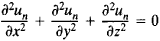# Solid Spherical Harmonic

The following article is from The Great Soviet Encyclopedia (1979). It might be outdated or ideologically biased.

## Solid Spherical Harmonic

A solid spherical harmonic of degree n is a homogeneous function un in the rectangular coordinates x, y, and z that satisfies Laplace’s equation:There are 2n + 1 linearly independent homogeneous polynomials in x, y, and z of integral positive degree n that are solid spherical harmonics. Their linear combination represents the general form of such a polynomial of degree n. For example, the general forms of homogeneous polynomials of degree 0, 1, and 2 that are solid spherical harmonics are represented by

u0 = a

u1 = axby + cz

u2 = a(x2z2) + b(y2z2) + cxy + dyz + ezx

where a, b, c, d, and e are arbitrary constants.

If the spherical coordinates r, θ, and φ are introduced instead of the rectangular coordinates x, y, and z, then solid spherical harmonics may be expressed in terms of the spherical surface harmonics Yn(θ, φ) according to the formula

un = rnYn(θ, φ)

To every solid spherical harmonic un of degree n there corresponds a solid spherical harmonic r– 2n–1un of degree – n – 1.

Solid spherical harmonics are used in finding the general solution of Laplace’s equation and in solving problems of mathematical physics for regions bounded by spherical surfaces.